RIGHT TRIANGLE TRIGONOMETRYLearning Objectives - This is what you must know after studying the lecture and doing the practice problems!

1.   Find the angle of depression.
2.   Find the angle of elevation
.
3.   Find the bearing
.
4.
Solve right triangles.Now that we know how to find angles when given trigonometric ratios, we will use trigonometry to model and solve a few application problems.

Angle of Depression - see #1 in the "Examples" document

When we look at something below our location, the angle between the line of sight and the horizontal is called the Angle of Depression. In this case, the line of sight is depressed below the horizontal.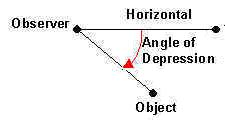Angle of Elevation - see #2 in the "Examples" document

When we look at something above our location, the angle between the line of sight and the horizontal is called the Angle of Elevation. In this case, the line of sight is elevated above the horizontal.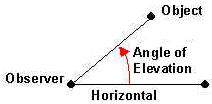Bearing - see #3 in the "Examples" document

In surveying and navigation, directions are generally given in terms of bearings. That is, an acute angle is given together with a compass heading. It is understood that the bearing is measured from a fixed north-south line to an east-west line. These lines can be thought of as a rectangular coordinate system where the vertex of the angle is placed at the origin which is the intersection of the two lines.Examples:

Given is the bearing of S 35o E which is pronounced South 35o East. "South" tells you that the initial side of the angle is the negative y-axis and "East" tells you that the terminal side of the angle will be east of the y-axis or in the fourth quadrant!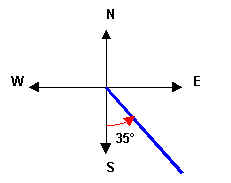Given is the bearing of N 70o W which is pronounced North 70o West. "North" tells you that the initial side of the angle is the positive y-axis and "West" tells you that the terminal side of the angle will be west of the y-axis or in the second quadrant!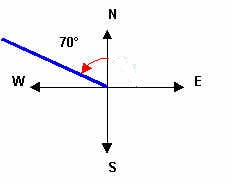Solving Right Triangles - see #4 and 5 in the "Examples" document

The process of finding the missing measurements in a triangle is known as "solving the triangle". If the triangle is a right triangle, then one of the angles is 90°. Therefore, you can solve the right triangle if you are given the measures of two of the three sides or if you are given the measure of one side and one of the other two angles.

We are going to solve right triangles by using the Pythagorean Theorem and the definitions of the trigonometric ratios.

We learned that the sides of a right triangle are related via the Pythagorean Theorem which stateswhere c is the length of the hypotenuse and a and b are the length of the legs.

Previously, we defined the six trigonometric ratios for some anglein a right triangle as follows: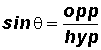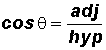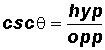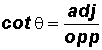In the formulas, we used the following abbreviation:

adj - the side of a right triangle adjacent to the angleopp - the side of a right triangle opposite the anglehyp - the hypotenuse of a right triagle

Memorization Aid for the Sine, Cosine, and Tangent:

SOHCAHTOA (sine equals opposite over hypotenuse; cosine equals adjacent over hypotenuse; and tangent equals opposite over adjacent).